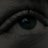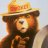# Hash Ribbons Indicator from Trading view

R

#### Ramesh16

##### Member
VIP
Can someone help to convert a Hash Ribbons indicator from Trading view to TOS-

Here is the code-

Code:
``````//@version=4
study("Hash Ribbons",overlay= false)

// NOTES

// The "Spring" is the confirmed Miner capitulation period:
// - The 1st "gray" circle is the start of Capitulation (1 month Hash Rate crosses UNDER 2 month Hash Rate)
// - Last "green" circle is the end of Capitulation (1 month Hash Rate crosses OVER 2 month Hash Rate)
// - The "greener" the spring gets (up until blue) represents Hash Rate recovery (it is increasing)
// - The "blue" circle is the first instance of positive momentum following recovery of Hash Rate (1m HR > 2m HR). This is historically a rewarding place to buy with limited downside.

// INPUTS

type = input('Ribbons',options=['Ribbons','Oscillator'],title="Plot Type")
len_s = input(30,"Hash Rate Short SMA (days).")
len_l = input(60,"Hash Rate Long SMA (days).")
signals = input(true, "Plot Signals")
plot_halvings = input(true,"Plot Halvings")
raw = input(false, "Plot Raw Hash Rate")

// HASH RATE MA

// HR on TV only has "yesterday's" value --> use "lookahead_on" when running live (on current bar), to pull forward yesterdays data

// DAILY TIMEFRAME MGMT

is_newbar(res) =>
t = time(res) // res calculated below \/
change(t) != 0 ? true : false

// Check how many bars are in our upper (otf) timeframe
since_new_bar = barssince(is_newbar("D")) //1-360 for minutes, D = Daily, W = Weekly, M = Monthly
D_total_bars = int(na)
D_total_bars := since_new_bar == 0 ? since_new_bar : D_total_bars // calculates the total number of current time frame bars in the OTF

// INDICATORS

HR_short = float(na)
HR_long = float(na)
HR_raw = float(na)
s10 = float(na)
s20 = float(na)

HR_short := barstate.isrealtime ? live_HR_short : hist_HR_short
HR_long := barstate.isrealtime ? live_HR_long : hist_HR_long
HR_raw := barstate.isrealtime ? live_HR_raw : hist_HR_raw

s10 := barstate.isrealtime ? (since_new_bar == D_total_bars ? daily_s10 : s10) : daily_s10
s20 := barstate.isrealtime ? (since_new_bar == D_total_bars ? daily_s20 : s20) : daily_s20

capitulation = crossunder(HR_short,HR_long)
miner_capitulation = HR_short<HR_long
recovering = HR_short > HR_short and HR_short > HR_short and HR_short > HR_short and miner_capitulation
recovered = crossover(HR_short,HR_long)

// HASH BOTTOM + PA SIGNAL

or
(s10>s20 and crossover(HR_short,HR_long))
)

// OSCILLATOR

delta = HR_short-HR_long
diff = (delta/HR_short)*100

// PLOT - DEFAULT

plot(raw ? HR_raw : na, color = color.green, linewidth = 1, style = plot.style_line, title='HR Raw')
p1=plot(type=='Ribbons'? HR_long : na, color = color.gray, linewidth = 2, style = plot.style_line,title='HR SMA Long')
p2=plot(type=='Ribbons'? HR_short : na, color = (HR_short<HR_long? color.red : color.lime), linewidth = 2, style = plot.style_line,title = 'HR SMA Short')
fill(p1,p2,color=(HR_short<HR_long?color.red:na),transp=30)

// PLOT - OSCILLATOR

plot(type=='Oscillator' ? diff : na,style=plot.style_columns,color=(diff<0?color.red:color.blue),title='Oscillator')

// PLOT - SIGNALS

plotshape(signals ? capitulation :na,style=shape.circle,location=location.top,color=color.gray,size=size.normal,transp=50,text='Capitulation',textcolor=color.black,title='Capitulation')
plotshape(signals ? miner_capitulation : na,style=shape.circle,location=location.top,color=color.green,size=size.normal,transp=90,title='Miner Capitulation')
plotshape(signals ? recovering : na,style=shape.circle,location=location.top,color=color.green,size=size.normal,transp=50,title='Recovering')
plotshape(signals ? recovered : na,style=shape.circle,location=location.top,color=color.lime,size=size.normal,transp=0,textcolor=color.white,title='Recovered')

// HALVINGS

halving_1 = timestamp(2012,11,28,0,0)
halving_2 = timestamp(2016,7,9,0,0)
halving_3 = timestamp(2020,5,12,0,0) // projected! https://www.bitcoinclock.com/
band = 2
h1_range = time >= halving_1 - band*(24*60*60*1000) and time <= halving_1 + band*(24*60*60*1000) //adds 3 day either side for chart visibility
h2_range = time >= halving_2 - band*(24*60*60*1000) and time <= halving_2 + band*(24*60*60*1000) //adds 3 day either side for chart visibility
h3_range = time >= halving_3 - band*(24*60*60*1000) and time <= halving_3 + band*(24*60*60*1000) //adds 3 day either side for chart visibility
bgcolor(h1_range and plot_halvings? color.red : na, transp = 20)
bgcolor(h2_range and plot_halvings? color.red : na, transp = 20)
bgcolor(h3_range and plot_halvings? color.red : na, transp = 20)Madrid Moving Average Ribbons for ThinkorSwim Indicators 5Midpoint Moving Average Indicator Questions 2